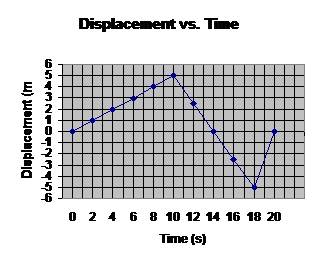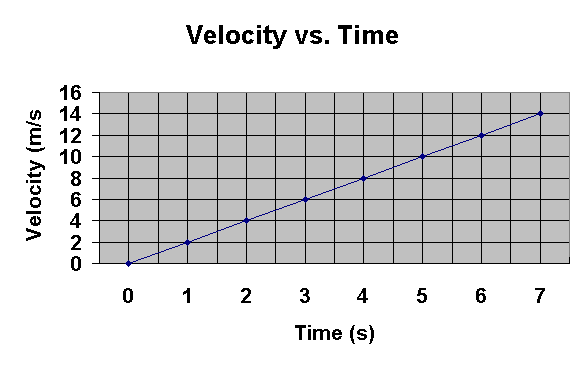Sample Test                                                           October 13, 2021

AP Physics                                                    Mr. Byrne

[Image_Link]image002.gif>a)      What is the distance traveled after 16 seconds?

b)      What is the displacement after 16 seconds?

c)      What is the velocity at t = 14 s?

d)      What is the acceleration at t = 19 s?

2) Sketch the displacement-time and acceleration-time graphs that describe the motion depicted in the following graph.  On each graph, indicate where zero on the y-axis is located.3)      Can an object having constant acceleration ever stop and stay stopped?

4)      Races are timed to 1/1000 of a second.  What distance could a roller blader moving at a speed of 10 m/s travel in that period of time?

5)      A bacterium swims with a speed of 3.5 micrometers/second.  How long would it take this bacterium to swim across a petri dish having a diameter of 8.4 cm?

6)      A speedboat increases in speed uniformly from 20 m/s to 30 m/s in a distance of 200 m.  Find the acceleration and the time it takes to travel this distance.

7)      If one object has a greater speed than a second object, does the first necessarily have a greater acceleration?  Explain, using examples.

8)      A car slows from 25 m/s to rest in 5 s.  How far did it travel in that time?

9)      A car traveling at 90 km/h strikes a tree.  The front end of the car compresses and the driver comes to rest after traveling 0.80 m.  What was the average acceleration of the driver during the collision?

10)   FOR YOU FORENSIC EXPERTS:  In coming to a stop, a car leaves skid marks 80 m long on the highway.  Assuming a deceleration of – 7.00 m/s2, estimate the speed of the car just before braking.

1. a) Distance is the total length of the path traveled. From 0 seconds to 10 seconds, the object traveled 5 m. From 10 s to 16 s, it traveled 7.5 m. The total distance is 12.5 m.

1. b) The displacement after 16 s is found directly from the graph. At t = 16 s, d = -2.5 m.

1. c) The slope of this graph represents the velocity. To find the slope at the t = 14 s point, chose any two points along the line segment that includes t = 14 s and use: slope = delta y/ delta x. I would choose (14, 0) and (10, 5). The slope is -5 m/ 4 s, which equals -1.25 m/s. This is the velocity.

1. d) At t = 19 s, the graph is a straight line, meaning velocity is constant. Therefore, acceleration is zero.

2. The displacement-time graph is a curve (because velocity changes) and it curves upward (like y = x2). The acceleration-time graph is a horizontal line (always for constant acceleration problems) and is positive (see the slope of the velocity-time graph).

3. No. If the acceleration remains constant, the instant after the object stops, it will begin to move in the direction of the acceleration.

4. Use d = average speed x time. Average speed = 10 m/s; t = 1 x 10-3. So, d = 1 x 10-2m, or 1 cm.

5. Use t = d / avg. velocity. Avg vel. = 3.5 micrometers/sec, which is 3.5 x 10-6m/s; d = 8.4 cm, which is 0.084 m. So, t = 24,000 s, or 6.7 hrs.

6. List the given information:

vi = 20 m/s;
vf = 30 m/s;
d = 200 m.
To find a, use vf2 = vi2 + 2ad.
Substitute with units. a = 1.25 m/s2.
To find t, use vf = vi + at. Substitute with units.
t = 8 s.

7. No. The faster object may be moving at constant speed (zero acceleration).

8. List the given information:

vi = 25 m/s;
vf = 0 m/s;
t = 5 s.
To find d, use d = 1/2(vi + vf)t. Substitute with units.
d = 62.5 m.

9. List the given information:

vi = 90 km/h, which is 25 m/s;
vf = 0 m/s;
d = 0.80 m.
To find a, use vf2 = vi2 + 2ad. Substitute with units.
a = -390.6 m/s2. Note that this is a very high acceleration. It is so high, the human body could not withstand it and would be destroyed.

10. List the given information:

a = -7.00 m/s2;
vf = 0 m/s;
d = 80 m.
To find vi, use vf2 = vi2 + 2ad.
vi = 33.5 m/s.